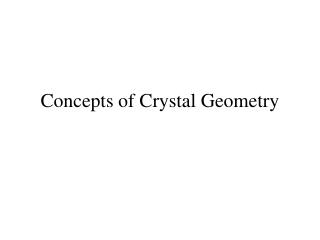DownloadDownload PresentationConcepts of Crystal Geometry

# Concepts of Crystal Geometry

Download Presentation## Concepts of Crystal Geometry

- - - - - - - - - - - - - - - - - - - - - - - - - - - E N D - - - - - - - - - - - - - - - - - - - - - - - - - - -
##### Presentation Transcript

1. Concepts of Crystal Geometry

2. X-ray diffraction analysis shows that the atoms in a metal • crystal are arranged in a regular, repeated three-dimensional • pattern. • The most elementary crystal structure is the simple cubic lattice • (Fig. 9-1). Figure 9-1 Simple cubic structure.

3. We now introduce atoms and molecules, or “repeatable • structural units”. • The unit cell is the smallest repetitive unit that there are 14 • space lattices. • These lattices are based on the seven crystal structures. • The points shown in Figure 9-1 correspond to atoms or groups • of atoms. • The 14 Bravis lattices can represent the unit cells for all • crystals.

4. Figure 9-2 (a) The 14 Bravais space lattices (P = primitive or simple; I = body-centered cubic; F = face-center cubic; C = base-centered cubic

5. Figure 9-2(b)

6. Figure 9-2(c)

7. Figure 9-2(d)

8. Figure 9-2(e)

9. Figure 9-2(f)

10. Figure 9-3 a) Body-centered cubic structure; b) face-centered cubic structure.

11. Figure 9-4 Hexagonal close-packed structure Figure 9-5 Stacking of close-packed spheres.

12. Three mutually perpendicular axes are arbitrarily placed through one of the corners of the cell. • Crystallographic planes and directions will be specified with respect to these axes in terms of Miller indices. • A crystallographic plane is specified in terms of the length of its intercepts on the three axes, measured from the origin of the coordinate axes. • To simplify the crystallographic formulas, the reciprocals of these intercepts are used. • They are reduced to a lowest common denominator to give the Miller indices (hkl) of the plane.

13. For example, the plane ABCD in Fig. 9-1 is parallel to the • x and z axes and intersects the y axis at one interatomic • distance ao. Therefore, the indices of the plane are • , or (hkl)=(010). Figure 9-1 Simple cubic structure.

14. There are six crystallographically equivalents planes of • the type (100). • Any one of which can have the indices (100), (010), • (001), depending upon the choice of • axes. • The notation {100} is used when they are to be considered • as a group,or family of planes.

15. Figure 9.6(a)shows another plane and its intercepts. Figure 9-6(a) Indexing of planes by Miller indices rules in the cubic unit cell

16. As usual, we take the inverse of the intercepts and multiply them by their common denominator so that we end up with integers. In Figure 9.6(a), we have

17. Figure 9.6(b) shows an indeterminate situation. Thus, we have to translate the plane to the next cell, or else translate the origin. Figure 9-6(b) Another example of indexing of planes by Miller rules in the cubic unit cell.